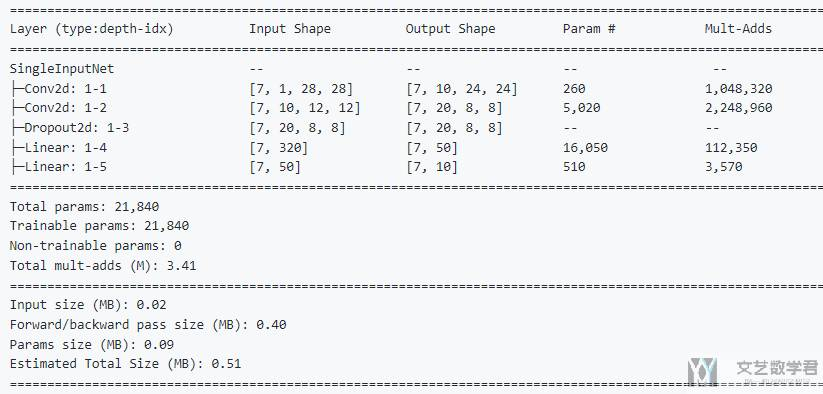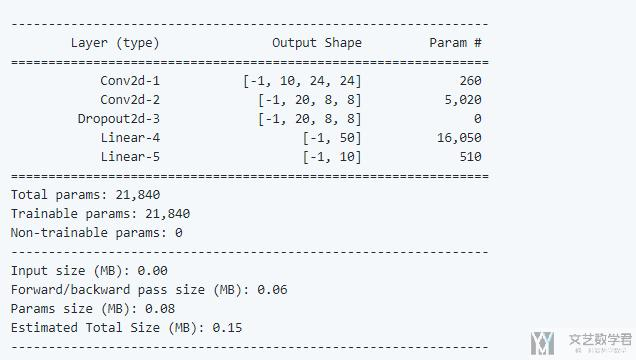# Pytorch模型概览(Pytorch Summary)2020年4月8日07:33:33

1 1814字阅读6分2秒

## 简介

【更新】目前官方已经推荐使用另外一个库，Github-torchinfo，来完成相同的功能。如果想要看到模型的结构的可视化，可以直接写入 tensorboard，参考这个链接，在 Pytorch 中使用 Tensorboard 进行可视化

## Torchinfo 简单使用介绍

1. pip install torchinfo

1. from torchinfo import summary
2. model = ConvNet()
3. batch_size = 16
4. summary(model, input_size=(batch_size, 1, 28, 28))

1. input_data = torch.randn(1, 300)
2. other_input_data = torch.randn(1, 300).long()
3. model = MultipleInputNetDifferentDtypes()
4. summary(model, input_data=[input_data, other_input_data, ...])## Pytorch Summary 简单使用介绍

1. pip install torchsummary

1. from torchsummary import summary
2. summary(your_model, input_size=(channels, H, W))

1. import torch
2. import torch.nn as nn
3. import torch.nn.functional as F
4. from torchsummary import summary
5. class Net(nn.Module):
6.     def __init__(self):
7.         super(Net, self).__init__()
8.         self.conv1 = nn.Conv2d(1, 10, kernel_size=5)
9.         self.conv2 = nn.Conv2d(10, 20, kernel_size=5)
10.         self.conv2_drop = nn.Dropout2d()
11.         self.fc1 = nn.Linear(320, 50)
12.         self.fc2 = nn.Linear(50, 10)
13.     def forward(self, x):
14.         x = F.relu(F.max_pool2d(self.conv1(x), 2))
15.         x = F.relu(F.max_pool2d(self.conv2_drop(self.conv2(x)), 2))
16.         x = x.view(-1, 320)
17.         x = F.relu(self.fc1(x))
18.         x = F.dropout(x, training=self.training)
19.         x = self.fc2(x)
20.         return F.log_softmax(x, dim=1)
21. device = torch.device("cuda" if torch.cuda.is_available() else "cpu") # PyTorch v0.4.0
22. model = Net().to(device)
23. summary(model, (1, 28, 28))• 微信公众号
• 关注微信公众号
•• QQ群
• 我们的QQ群号
•• 本文由 发表于 2020年4月8日07:33:33
• 转载请务必保留本文链接：https://mathpretty.com/12052.html